## Section Exercises

1. Define an ellipse in terms of its foci.

2. Where must the foci of an ellipse lie?

3. What special case of the ellipse do we have when the major and minor axis are of the same length?

4. For the special case mentioned above, what would be true about the foci of that ellipse?

5. What can be said about the symmetry of the graph of an ellipse with center at the origin and foci along the y-axis?

For the following exercises, determine whether the given equations represent ellipses. If yes, write in standard form.

6. $2{x}^{2}+y=4$

7. $4{x}^{2}+9{y}^{2}=36$

8. $4{x}^{2}-{y}^{2}=4$

9. $4{x}^{2}+9{y}^{2}=1$

10. $4{x}^{2}-8x+9{y}^{2}-72y+112=0$

For the following exercises, write the equation of an ellipse in standard form, and identify the end points of the major and minor axes as well as the foci.

11. $\frac{{x}^{2}}{4}+\frac{{y}^{2}}{49}=1$

12. $\frac{{x}^{2}}{100}+\frac{{y}^{2}}{64}=1$

13. ${x}^{2}+9{y}^{2}=1$

14. $4{x}^{2}+16{y}^{2}=1$

15. $\frac{{\left(x - 2\right)}^{2}}{49}+\frac{{\left(y - 4\right)}^{2}}{25}=1$

16. $\frac{{\left(x - 2\right)}^{2}}{81}+\frac{{\left(y+1\right)}^{2}}{16}=1$

17. $\frac{{\left(x+5\right)}^{2}}{4}+\frac{{\left(y - 7\right)}^{2}}{9}=1$

18. $\frac{{\left(x - 7\right)}^{2}}{49}+\frac{{\left(y - 7\right)}^{2}}{49}=1$

19. $4{x}^{2}-8x+9{y}^{2}-72y+112=0$

20. $9{x}^{2}-54x+9{y}^{2}-54y+81=0$

21. $4{x}^{2}-24x+36{y}^{2}-360y+864=0$

22. $4{x}^{2}+24x+16{y}^{2}-128y+228=0$

23. $4{x}^{2}+40x+25{y}^{2}-100y+100=0$

24. ${x}^{2}+2x+100{y}^{2}-1000y+2401=0$

25. $4{x}^{2}+24x+25{y}^{2}+200y+336=0$

26. $9{x}^{2}+72x+16{y}^{2}+16y+4=0$

For the following exercises, find the foci for the given ellipses.

27. $\frac{{\left(x+3\right)}^{2}}{25}+\frac{{\left(y+1\right)}^{2}}{36}=1$

28. $\frac{{\left(x+1\right)}^{2}}{100}+\frac{{\left(y - 2\right)}^{2}}{4}=1$

29. ${x}^{2}+{y}^{2}=1$

30. ${x}^{2}+4{y}^{2}+4x+8y=1$

31. $10{x}^{2}+{y}^{2}+200x=0$

For the following exercises, graph the given ellipses, noting center, vertices, and foci.

32. $\frac{{x}^{2}}{25}+\frac{{y}^{2}}{36}=1$

33. $\frac{{x}^{2}}{16}+\frac{{y}^{2}}{9}=1$

34. $4{x}^{2}+9{y}^{2}=1$

35. $81{x}^{2}+49{y}^{2}=1$

36. $\frac{{\left(x - 2\right)}^{2}}{64}+\frac{{\left(y - 4\right)}^{2}}{16}=1$

37. $\frac{{\left(x+3\right)}^{2}}{9}+\frac{{\left(y - 3\right)}^{2}}{9}=1$

38. $\frac{{x}^{2}}{2}+\frac{{\left(y+1\right)}^{2}}{5}=1$

39. $4{x}^{2}-8x+16{y}^{2}-32y - 44=0$

40. ${x}^{2}-8x+25{y}^{2}-100y+91=0$

41. ${x}^{2}+8x+4{y}^{2}-40y+112=0$

42. $64{x}^{2}+128x+9{y}^{2}-72y - 368=0$

43. $16{x}^{2}+64x+4{y}^{2}-8y+4=0$

44. $100{x}^{2}+1000x+{y}^{2}-10y+2425=0$

45. $4{x}^{2}+16x+4{y}^{2}+16y+16=0$

For the following exercises, use the given information about the graph of each ellipse to determine its equation.

46. Center at the origin, symmetric with respect to the x– and y-axes, focus at $\left(4,0\right)$, and point on graph $\left(0,3\right)$.

47. Center at the origin, symmetric with respect to the x– and y-axes, focus at $\left(0,-2\right)$, and point on graph $\left(5,0\right)$.

48. Center at the origin, symmetric with respect to the x– and y-axes, focus at $\left(3,0\right)$, and major axis is twice as long as minor axis.

49. Center $\left(4,2\right)$ ; vertex $\left(9,2\right)$ ; one focus: $\left(4+2\sqrt{6},2\right)$ .

50. Center $\left(3,5\right)$ ; vertex $\left(3,11\right)$ ; one focus: $\left(3,\text{ 5+4}\sqrt{\text{2}}\right)$

51. Center $\left(-3,4\right)$ ; vertex $\left(1,4\right)$ ; one focus: $\left(-3+2\sqrt{3},4\right)$

For the following exercises, given the graph of the ellipse, determine its equation.

52.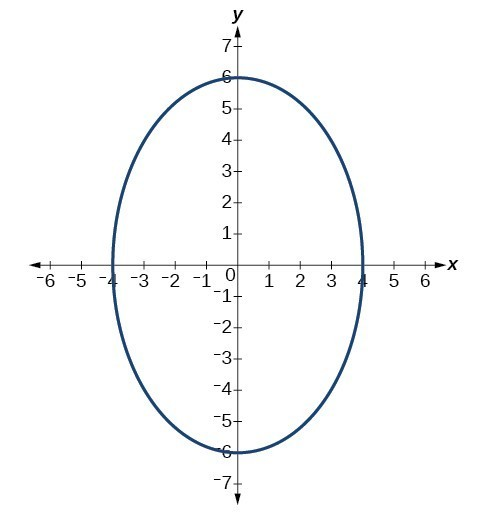53.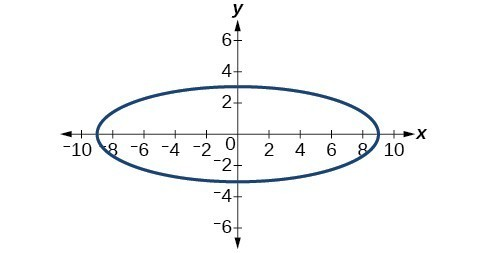54.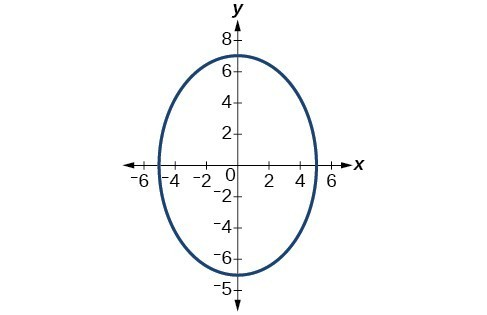55.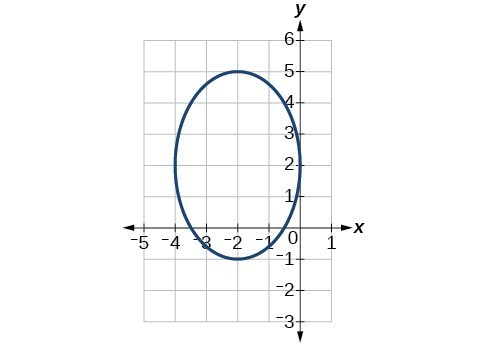56.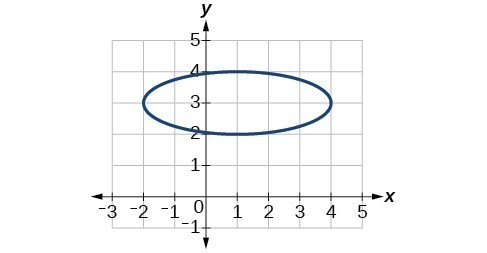For the following exercises, find the area of the ellipse. The area of an ellipse is given by the formula $\text{Area}=a\cdot b\cdot \pi$.

57. $\frac{{\left(x - 3\right)}^{2}}{9}+\frac{{\left(y - 3\right)}^{2}}{16}=1$

58. $\frac{{\left(x+6\right)}^{2}}{16}+\frac{{\left(y - 6\right)}^{2}}{36}=1$

59. $\frac{{\left(x+1\right)}^{2}}{4}+\frac{{\left(y - 2\right)}^{2}}{5}=1$

60. $4{x}^{2}-8x+9{y}^{2}-72y+112=0$

61. $9{x}^{2}-54x+9{y}^{2}-54y+81=0$

62. Find the equation of the ellipse that will just fit inside a box that is 8 units wide and 4 units high.

63. Find the equation of the ellipse that will just fit inside a box that is four times as wide as it is high. Express in terms of $h$, the height.

64. An arch has the shape of a semi-ellipse (the top half of an ellipse). The arch has a height of 8 feet and a span of 20 feet. Find an equation for the ellipse, and use that to find the height to the nearest 0.01 foot of the arch at a distance of 4 feet from the center.

65. An arch has the shape of a semi-ellipse. The arch has a height of 12 feet and a span of 40 feet. Find an equation for the ellipse, and use that to find the distance from the center to a point at which the height is 6 feet. Round to the nearest hundredth.

66. A bridge is to be built in the shape of a semi-elliptical arch and is to have a span of 120 feet. The height of the arch at a distance of 40 feet from the center is to be 8 feet. Find the height of the arch at its center.

67. A person in a whispering gallery standing at one focus of the ellipse can whisper and be heard by a person standing at the other focus because all the sound waves that reach the ceiling are reflected to the other person. If a whispering gallery has a length of 120 feet, and the foci are located 30 feet from the center, find the height of the ceiling at the center.

68. A person is standing 8 feet from the nearest wall in a whispering gallery. If that person is at one focus, and the other focus is 80 feet away, what is the length and height at the center of the gallery?